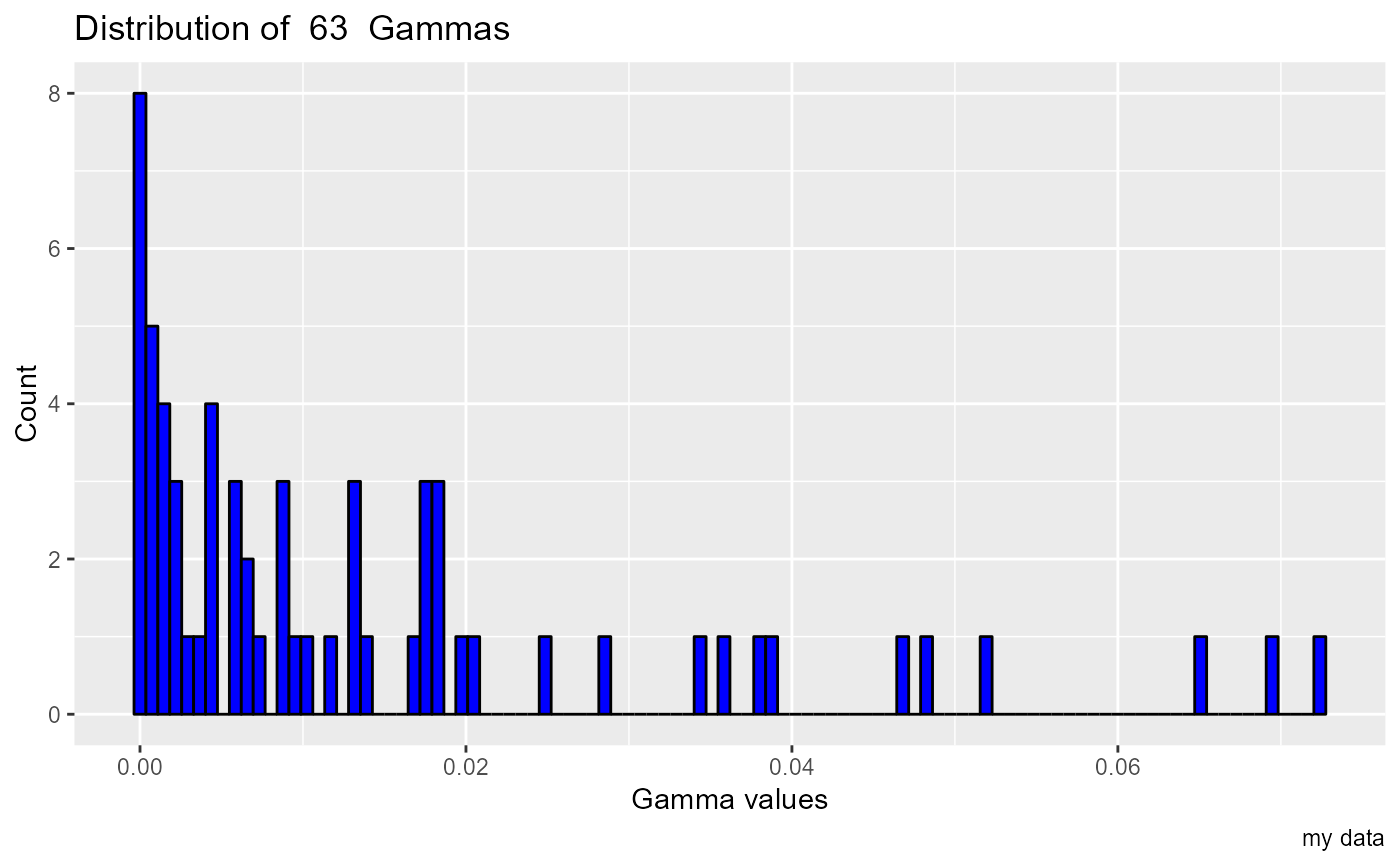Produces a histogram showing the distribution in a population of Gamma values, used to examine the result of a full embedding search. Pass the result of fe_search() to this function to look for structure in the predictors. For example, it this histogram is bimodal, there is probably one input variable which is absolutely required for a good predictive function, so the histogram divides into the subset containing that variable, and the others that don't.

## Usage

gamma_histogram(fe_results, bins = 100, caption = "")

## Arguments

fe_results

The result of fe_search or full_embedding_search. A matrix containing a column labeled Gamma, of Numeric Gamma values. It also contains an integer column of masks, but that is not used by this function.

bins

Numeric, number of bins in the histogram

caption

Character string caption for the plot

## Value

a ggplot object, a histogram showing the distribution of Gamma values full embedding search output

## Examples

e6 <- embed(mgls, 7)
t <- e6[ ,1]
p <- e6[ ,2:7]
full_search <- fe_search(predictors = p, target = t)
gamma_histogram(full_search, caption = "my data")